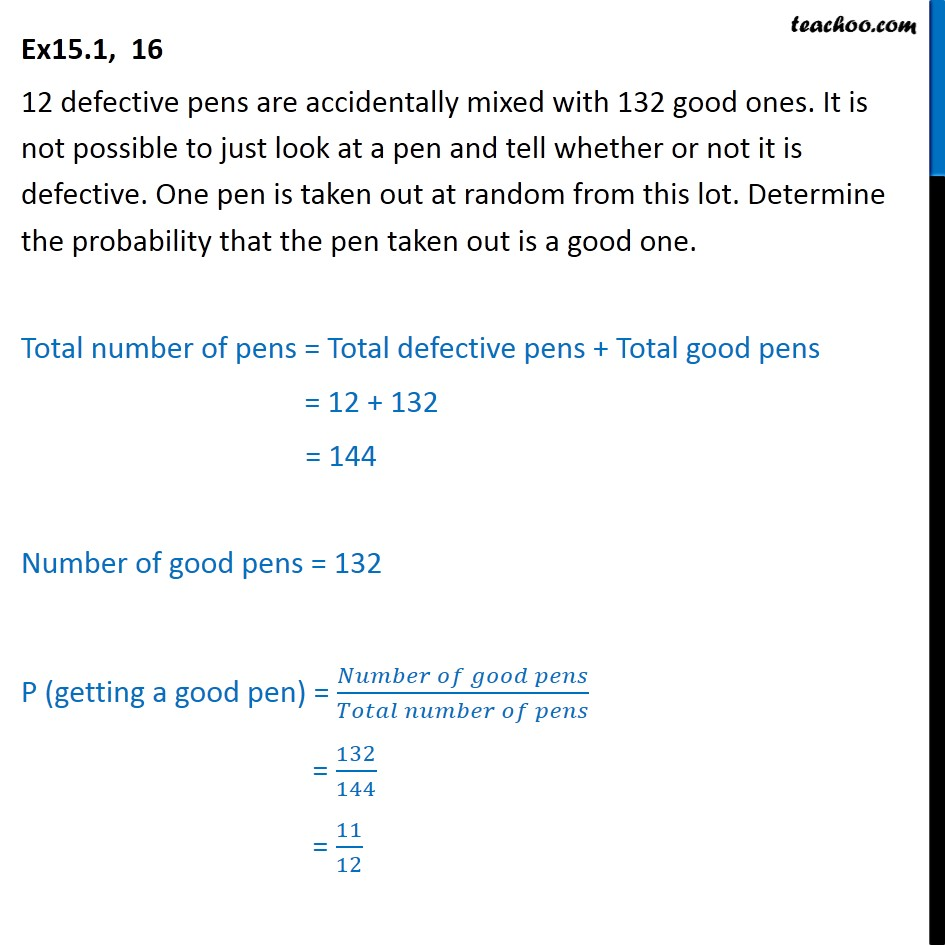1. Chapter 15 Class 10 Probability (Term 1)
2. Serial order wise
3. Ex 15.1

Transcript

Ex15.1, 16 12 defective pens are accidentally mixed with 132 good ones. It is not possible to just look at a pen and tell whether or not it is defective. One pen is taken out at random from this lot. Determine the probability that the pen taken out is a good one. Total number of pens = Total defective pens + Total good pens = 12 + 132 = 144 Number of good pens = 132 P (getting a good pen) = ( )/( ) = 132/144 = 11/12